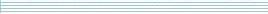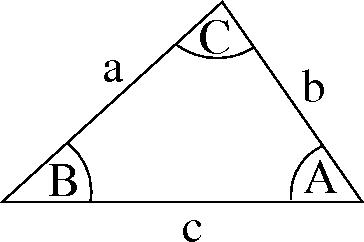| home      | people     | research     | publications    | seminars    | events     | contactCommunication Networks   | Systems Biology   | Hybrid Systems    | Machine Learning   | Dynamics & Interaction### Schools Mathematics Grand Challenge

PROBLEM 25:

An office block contains a very long corridor, containing 100 doors in a row, numbered from 1 to 100 inclusive. By a very odd coincidence, there are also 100 people working along this corridor. One day, one of these people comes into the corridor and closes all of the doors. After this person has left, a second person enters the corridor and, starting with door 2, opens every second door.

After this is completed, a third person comes along and, starting at door 3, checks every third door, opening it if it is closed, and closing it if it is open. After this, a fourth person comes along, and checks every fourth door, opening it if it is closed and closing it if it is open. This continues for the 5th, 6th, 7th person and so on until the 100th person comes and checks the 100th door, opening it if it is closed and closing it if it is open. (The 5th person checks every 5th door, the 6th person checks every 6th door and so on.)

After the 100th person has come and left, how many of the 100 doors on the corridor are closed?

NOTE: The 3rd person will check doors number 3, 6, 9, ... up to door 99 and then leave. Similarly, the 7th person checks doors 7, 14, 21, ... up to door 98 and then leaves and so on.

PROBLEM 26:

How many positive whole numbers have the following two properties:

• No digit of the number is 0;
• The sum of the digits of the number is equal to 9?
• For example, 9, 18, 162 and 261 are ok but 108 and 2061 are not.

PROBLEM 27:

In this problem we adopt the convention that the lengths of the sides of a triangle are labelled a, b, c and the angles opposite each side are labelled A, B, C, as in the diagram below.In a triangle for which,

b^2 + c^2 = a^2 + (b x c).

what is the size (in degrees) of the angle A? (Here the notation b x c means b multiplied by c and a^2 means a squared or a x a.)

HINT: You may need the Theorem of Pythagoras for this. In a triangle with a right angle like that shown below, d^2 = e^2 + f^2.